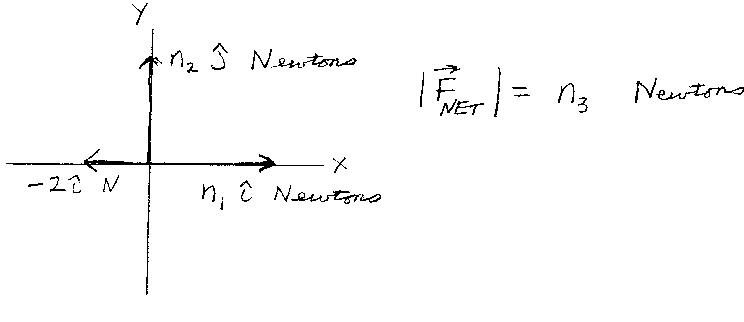Problem A1: Consider the three forces shown in the figure. One force is equal to F1 = -2 i Newtons. A second force is equal to F2 = n1 i Newtons. A third force is equal to F3 = n2 j Newtons. If all three forces act at once, what is the magnitude of the resultant "net" force. That is, if the magnitude of the net force |Fnet| = n3 Newtons, what is n3?n1 = n2 = Input n3:

If you are currently in my class, you can record your grade by entering your name and student ID number (without the leading zeros) below and clicking on "record grade".
 First Name = Last Name = ID = Problem: